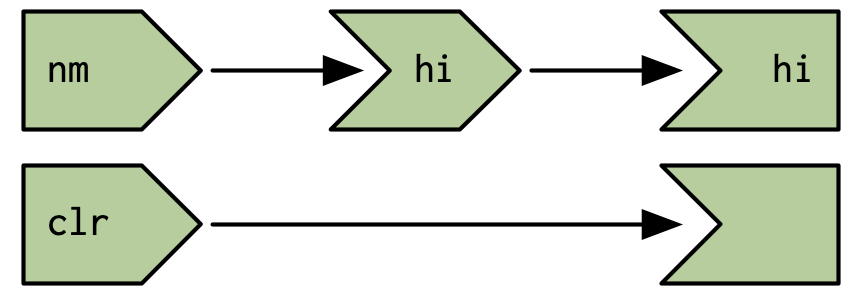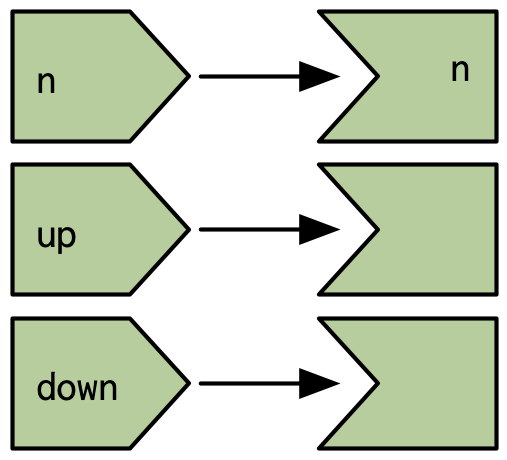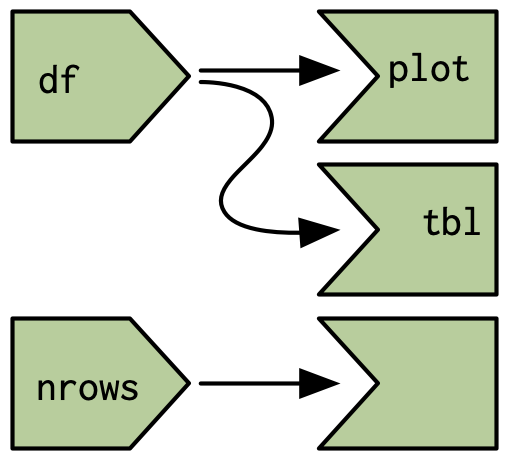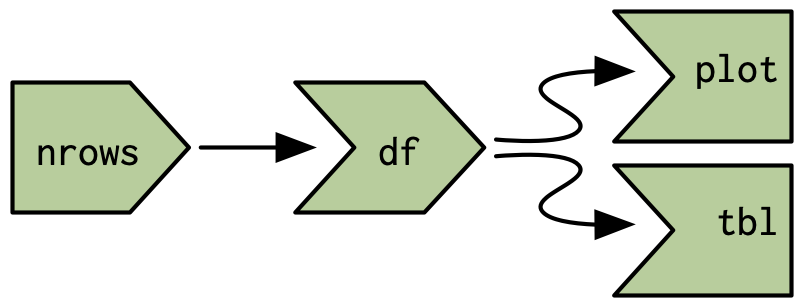16 Escaping the graph

16.1 Introduction

Shiny’s reactive programming framework is incredibly useful because it automatically determines the minimal set of computations needed to update all outputs when an input changes. But this framework is deliberately constraining, and sometimes you need to break free to do something risky but necessary.

In this chapter you’ll learn how you can combine reactiveValues() and observe()/observeEvent() to connect the right hand side of the reactive graph back to the left hand side. These techniques are powerful because they give you manual control over parts of the graph. But they’re also dangerous because they allow your app to do unnecessary work. Most importantly, you can now create infinite loops where your app gets stuck in a cycle of updates that never ends.

If you find the ideas explored in this chapter to be interesting, you might also want to look at the shinySignals and rxtools packages. These are both experimental packages, designed to explore “higher order” reactivity, reactives that are created programmatically from other reactives. I wouldn’t recommend you use them in “real” apps, but reading the source code might be illuminating.

16.2 What doesn’t the reactive graph capture?

In Section 14.4 we discussed what happens when the user causes an input to be invalidated. There are two other important cases where you as the app author might invalidate an input:

• You call an update function setting the value argument. This sends a message to the browser to change the value of an input, which then notifies R that the input value has been changed.

• You modify the value of a reactive value (created with reactiveVal() or reactiveValues()).

It’s important to understand that in both of these cases a reactive dependency is not created between the reactive value and the observer. While these actions cause the graph to invalidate, they are not recorded through new connections53.

To make this idea concrete, take the following simple app, with reactive graph shown in Figure 16.1.

ui <- fluidPage(
textInput("nm", "name"),
actionButton("clr", "Clear"),
textOutput("hi")
)
server <- function(input, output, session) {
hi <- reactive(paste0("Hi ", input\$nm))
output\$hi <- renderText(hi())
observeEvent(input\$clr, {
updateTextInput(session, "nm", value = "")
})
}Figure 16.1: The reactive graph does not record the connection between the unnamed observer and the nm input; this dependency is outside of its scope.

What happens when you press the clear button?

1. input\$clr invalidates, which then invalidates the observer.
2. The observer recomputes, recreating the dependency on input\$clr, and telling the browser to change the value of the input control.
3. The browser changes the value of nm.
4. input\$nm invalidates, invalidating hi() then output\$hi.
5. output\$hi recomputes, forcing hi() to recompute.

None of these actions change the reactive graph, so it remains as in Figure 16.1 and the graph does not capture the connection from the observer to input\$nm.

16.3 Case studies

Next, lets take a look at a few useful cases where you might combine reactiveValues() and observeEvent() or observe() to solve problems that are otherwise very challenging (if not impossible). These are useful templates for your own apps.

16.3.1 One output modified by multiple inputs

To get started we’ll tackle a very simple problem: I want a common text box that’s updated by multiple events54.

ui <- fluidPage(
actionButton("drink", "drink me"),
actionButton("eat", "eat me"),
textOutput("notice")
)
server <- function(input, output, session) {
r <- reactiveValues(notice = "")
observeEvent(input\$drink, {
r\$notice <- "You are no longer thirsty"
})
observeEvent(input\$eat, {
r\$notice <- "You are no longer hungry"
})
output\$notice <- renderText(r\$notice)
}

Things get slightly more complicated in the next example, where we have an app with two buttons that let you increase and decrease values. We use a reactiveValues() to store the current value, and then use observeEvent() to increment and decrement the value when the appropriate button is pushed. The main additional complexity here is that the new value of r\$n depends on the previous value.

ui <- fluidPage(
actionButton("up", "up"),
actionButton("down", "down"),
textOutput("n")
)
server <- function(input, output, session) {
r <- reactiveValues(n = 0)
observeEvent(input\$up, {
r\$n <- r\$n + 1
})
observeEvent(input\$down, {
r\$n <- r\$n - 1
})

output\$n <- renderText(r\$n)
}

Figure 16.2 shows the reactive graph for this example. Again note that the reactive graph does not include any connection from the observers back to the reactive value n.Figure 16.2: The reactive graph does not capture connections from observers to input values

16.3.2 Accumulating inputs

It’s a similar pattern if you want to accumulate data in order to support data entry. Here the main difference is that we use updateTextInput() to reset the text box after the user clicks the add button.

ui <- fluidPage(
textInput("name", "name"),
textOutput("names")
)
server <- function(input, output, session) {
r <- reactiveValues(names = character())
r\$names <- c(input\$name, r\$names)
updateTextInput(session, "name", value = "")
})

output\$names <- renderText(r\$names)
}

We could make this slightly more useful by providing a delete button and making sure that the add button doesn’t create duplicate names:

ui <- fluidPage(
textInput("name", "name"),
actionButton("del", "delete"),
textOutput("names")
)
server <- function(input, output, session) {
r <- reactiveValues(names = character())
r\$names <- union(r\$names, input\$name)
updateTextInput(session, "name", value = "")
})
observeEvent(input\$del, {
r\$names <- setdiff(r\$names, input\$name)
updateTextInput(session, "name", value = "")
})

output\$names <- renderText(r\$names)
}

16.3.3 Pausing animations

Another common use case is to provide a start and stop button that lets you control some recurring event. This example uses a running reactive value to control whether or not the number increments, and invalidateLater() to ensure that the observer is invalidated every 250 ms when running.

ui <- fluidPage(
actionButton("start", "start"),
actionButton("stop", "stop"),
textOutput("n")
)
server <- function(input, output, session) {
r <- reactiveValues(running = FALSE, n = 0)

observeEvent(input\$start, {
r\$running <- TRUE
})
observeEvent(input\$stop, {
r\$running <- FALSE
})

observe({
if (r\$running) {
r\$n <- isolate(r\$n) + 1
invalidateLater(250)
}
})
output\$n <- renderText(r\$n)
}

Notice in this case we can’t easily use observeEvent() because we perform different actions depending on whether running() is TRUE or FALSE. Since we can’t use observeEvent(), we must use isolate() — if we don’t this observer would also take a reactive dependency on n, which it updates, so it would get stuck in an infinite loop.

Hopefully these examples start to give you a flavour of what programming with reactiveValues() and observe() feels like. It’s very imperative: when this happens, do that; when that happens, do the other thing. This makes it easier to understand on a small scale, but harder to understand when bigger pieces start interacting. So generally, you’ll want to use this as sparingly as possible, and keep it isolated so that the smallest possible number of observers modify the reactive value.

16.3.4 Exercises

1. Provide a server function that draws a histogram of 100 random numbers from a normal distribution when normal is clicked, and 100 random uniforms.

ui <- fluidPage(
actionButton("rnorm", "Normal"),
actionButton("runif", "Uniform"),
plotOutput("plot")
)
2. Modify your code from above for to work with this UI:

ui <- fluidPage(
selectInput("type", "type", c("Normal", "Uniform")),
actionButton("go", "go"),
plotOutput("plot")
)
3. Rewrite your code from the previous answer to eliminate the use of observe()/observeEvent() and only use reactive(). Why can you do that for the second UI but not the first?

16.4 Anti-patterns

Once you get the hang of this pattern it’s easy to fall into bad habits:

server <- function(input, output, session) {
r <- reactiveValues(df = cars)
observe({
})

output\$plot <- renderPlot(plot(r\$df))
output\$table <- renderTable(r\$df)
}

In this simple case, this code doesn’t do much extra work compared to the alternative that uses reactive():

server <- function(input, output, session) {

output\$plot <- renderPlot(plot(df()))
output\$table <- renderTable(df())
}

But there are still two drawbacks:

• If the table or plot are in tabs that are not currently visible, the observer will still draw/plot them.

• If the head() throws an error, the observe() will terminate the app, but reactive() will propagate it so it’s displayed reactive throws an error, it won’t get propagated.

And things will get progressively worse as the app gets more complicated. It’s very easy to revert to the event-driven programming situation described in Section 13.2.3. You end up doing a lot of hard work to analyse the flow of events in your app, rather than relying on Shiny to handle it for you automatically.

It’s informative to compare the two reactive graphs. Figure 16.3 shows the graph from the first example. It’s misleading because it doesn’t look like nrows is connected to df(). Using a reactive, as in Figure 16.4, makes the precise connection easy to see. Having a reactive graph that is as simple as possible is important for both humans and for Shiny. A simple graph is easier for humans to understand, and a simple graph is easier for Shiny to optimise.Figure 16.3: Using reactive values and observers leaves part of the graph disconnectedFigure 16.4: Using a reactives makes the dependencies between the components very clear.

16.5 Summary

In the last four chapters, you have learned much more about the reactive programming model used by Shiny. You’ve learned why reactive programming is important (it allows Shiny to do just as much work as is required and no more), and the details of the reactive graph. You’ve also learned a bit about how the fundamental building blocks work under the hood, and how you can use them to escape the constraints of the reactive graph when needed.

The remainder of the book discusses Shiny through the lens of software engineering. In the next seven chapters, you’ll learn how to keep you Shiny apps maintainable, performant, and safe as they continue to grow in size and impact.